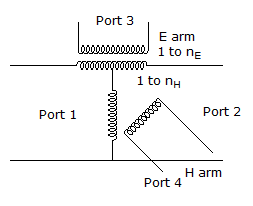# Electronics and Communication Engineering - Microwave Communication

### Exercise :: Microwave Communication - Section 4

11.

The two terms used to describe performance of a directional coupler are

 A. coupling and directivity B. gain and coupling C. gain and directivity D. gain and isolation

Explanation:

No answer description available for this question. Let us discuss.

12.

The number of PM radio channels which can be accommodated in a spectrum of 300 MHz is about

 A. 10 B. 100 C. 500 D. 1500

Explanation:

No answer description available for this question. Let us discuss.

13.

Consider the following statements

1. Gunn diode can be used in combinational logic circuits.
2. Gunn diode can be used in sequential logic circuits.
3. Both Gunn diode and GaAs Mesfet can be used in logic circuits.
Which of the above statements are correct?

 A. 1, 2, and 3 B. 1 and 2 only C. 2 and 3 only D. 1 and 3 only

Explanation:

No answer description available for this question. Let us discuss.

14.

In the given figure shows the equivalent circuit of a magic tee. If all ports are matchedA. nE = nH = 2 B. nE = nH = 0.707 C. nE = 2, nH = 0.707 D. nH = 0.707, nH = 2

Explanation:

No answer description available for this question. Let us discuss.

15.

Assertion (A): Microstrip is very commonly used in microwave integrated circuits.

Reason (R): Microstrip has an easy access to the top-surface so that active and passive discrete components can be easily mounted.

 A. Both A and R are correct and R is correct explanation of A B. Both A and R are correct but R is not correct explanation of A C. A is correct but R is wrong D. A is wrong but R is correct

Explanation:

A Microstrip line has a single dielectric substratc with ground plane on one side and a strip on the other face.